# Congruence subgroup

(diff) ← Older revision | Latest revision (diff) | Newer revision → (diff)

A subgroupof the general linear group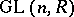over a ringwith the following property: There exists a non-zero two-sided idealofsuch that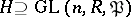, where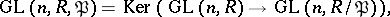that is,contains all matrices inthat are congruent to the unit matrix modulo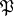. More generally, a subgroupof a linear groupof degreeoveris said to be a congruence subgroup iffor some non-zero two-sided ideal.

When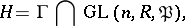is said to be the principal congruence subgroup corresponding to. The concept of a congruence subgroup arose first for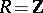. It is particularly effective and important from the point of view of applications for a Dedekind ringin the case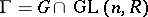, whereis an algebraic group defined over the field of fractions of.

How to Cite This Entry:
Congruence subgroup. Encyclopedia of Mathematics. URL: http://encyclopediaofmath.org/index.php?title=Congruence_subgroup&oldid=11756
This article was adapted from an original article by V.P. Platonov (originator), which appeared in Encyclopedia of Mathematics - ISBN 1402006098. See original article